Next: Constraints for zero-order terms Up: Continuity equation and local Previous: The NLO quasilocal functional

# Continuity equations in the four spin-isospin channels

Condition (48) sets constraints on the coupling constantsof the EDF. In our study, these explicit constraints were obtained, with the aid of the symbolic programming, as solutions of a linear system of equations, where each equation is found by considering the coefficients standing at a given product of pairs of secondary densities. Indices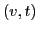correspond to the choice of the channel under examination: (0,0) for the CE in the scalar-isoscalar channel, (0,1) for the CE in the scalar-isovector channel, (1,0) for the CE in the vector-isoscalar channel, and (1,1) for the CE in the vector-isovector channel.

As a result of this analysis, for each spin-isospin channel we can classify the coupling constants in four categories defined in Ref. [Carlsson et al.(2008)Carlsson, Dobaczewski, and Kortelainen], namely, unrestricted, vanishing, independent, and dependent. The unrestricted coupling constants are not affected by condition (48) and vanishing ones are forced by this condition to be equal to zero. The remaining coupling constants obey sets of linear conditions, whereby one can express the dependent ones through independent ones. Obviously, for a given set of linear conditions, this can be done in very many different ways; below we present in each case only one choice thereof. We also use the name of a free coupling constant to denote either the unrestricted or independent one.

The structure of this section is as follows. First, in Table (1) we present for the four spin-isospin channels an overview of results by showing the number of unrestricted (U), vanishing (V), independent (I), and dependent (D) coupling constants of the EDF. The sum of the unrestricted and independent coupling constants gives the number of the free ones. Second, in Sec. 3.1 we discuss the simplest case of the zero-order terms, where one-body pseudopotentials reduce to simple potential functions, and we can link our results to those presented in the introductory Sec. 2.1. Next, in Sec. 3.2 we briefly describe the results obtained for the standard CE in the scalar-isoscalar channel. For the CEs in the three other spin-isospin channel, we present our results in Secs. 3.3-3.5, where, for clarity, only the second-order terms and general rules are discussed, while the results for fourth and sixth orders are collected in Appendices A-C.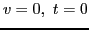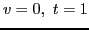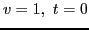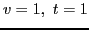Order Total U V I D U V I D U V I D U V I D 0 4 4 0 0 0 4 0 0 0 4 0 0 0 1 0 1 2 2 24 6 0 8 10 3 7 6 8 2 10 4 8 1 11 1 11 4 90 6 54 6 24 3 57 6 24 2 64 4 20 1 65 1 23 6 258 6 200 6 46 3 203 6 46 2 216 4 36 1 217 1 39 N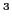LO 376 22 254 20 80 13 267 18 78 10 290 12 64 4 293 4 75

SubsectionsNext: Constraints for zero-order terms Up: Continuity equation and local Previous: The NLO quasilocal functional
Jacek Dobaczewski 2011-11-11